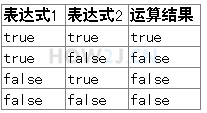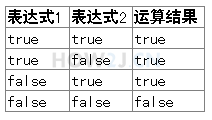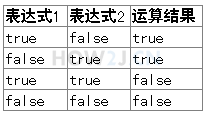# Java的算数操作符(八)

2022年4月18日 32点热度 0人点赞 0条评论

# 操作符系列教材 （一）- Java的算数操作符

 `+ - * / %`

 `++ --`

## 示例 1 : 基本算数操作符

 `+ - * /`

 `public` `class` `HelloWorld {` `    ``public` `static` `void` `main(String[] args) {` `        ``int` `i = ``10``;` `        ``int` `j = ``5``;` `        ``int` `a = i+j;` `        ``int` `b = i - j;` `        ``int` `c = i*j;` `        ``int` `d = i /j;` `    ``}` `}`

## 示例 2 : 任意运算单元的长度超过int

int a = 5;
long b = 6;
a+b -> 结果类型是long

 `public` `class` `HelloWorld {` `    ``public` `static` `void` `main(String[] args) {`   `        ``int` `a = ``5``;` `        ``long` `b = ``6``;` `        ``int` `c = (``int``) (a+b); ``//a+b的运算结果是long型，所以要进行强制转换` `        ``long` `d = a+b;` `        `  `    ``}` `}`

## 示例 3 : 任意运算单元的长度小于int

byte a = 1;
byte b= 2;
a+b -> int 类型

 `public` `class` `HelloWorld {` `    ``public` `static` `void` `main(String[] args) {` `        ``byte` `a = ``1``;` `        ``byte` `b= ``2``;` `        ``byte` `c = (``byte``) (a+b); ``//虽然a b都是byte类型，但是运算结果是int类型，需要进行强制转换` `        ``int` `d = a+b;` `    ``}` `}`

## 示例 4 : %取模

% 取余数，又叫取模
5除以2，余1

 `public` `class` `HelloWorld {` `    ``public` `static` `void` `main(String[] args) {`   `        ``int` `i = ``5``;` `        ``int` `j = ``2``;` `        ``System.out.println(i%j); ``//输出为1` `    ``}` `}`

## 示例 5 : 自增 自减

++
--

 `public` `class` `HelloWorld {` `    ``public` `static` `void` `main(String[] args) {`   `        ``int` `i = ``5``;` `        ``i++;` `        ``System.out.println(i);``//输出为6`   `    ``}` `}`

## 示例 6 : 自增 自减操作符置前以及置后的区别

int i = 5;
i++; 先取值，再运算
++i; 先运算，再取值

 `public` `class` `HelloWorld {` `    ``public` `static` `void` `main(String[] args) {` `        ``int` `i = ``5``;` `        ``System.out.println(i++); ``//输出5` `        ``System.out.println(i);   ``//输出6` `        `  `        ``int` `j = ``5``;` `        ``System.out.println(++j); ``//输出6` `        ``System.out.println(j);   ``//输出6` `    ``}` `}`

# 操作符系列教材 （二）- Java的关系操作符

> 大于
>= 大于或等于
< 小于
<= 小于或等于
== 是否相等
!= 是否不等

## 示例 1 : 关系操作符

> 大于
>= 大于或等于
< 小于
<= 小于或等于
== 是否相等
!= 是否不等

 `public` `class` `HelloWorld {` `    ``public` `static` `void` `main(String[] args) {` `       ``int` `a = ``5``;` `       ``int` `b = ``6``;` `       ``int` `c = ``5``;` `       `  `       ``System.out.println(a>b);  ``//返回 false` `       ``System.out.println(a>=c);  ``//返回 true` `       `  `       ``System.out.println(a==b); ``//返回false` `       ``System.out.println(a!=b);``//返回true` `       `  `}` `}`

# 操作符系列教材 （三）- Java的逻辑运算符

## 示例 1 : 长路与 和 短路与`public` `class` `HelloWorld {` `    ``public` `static` `void` `main(String[] args) {` `        ``//长路与  无论第一个表达式的值是true或者false,第二个的值，都会被运算` `        ``int` `i = ``2``;` `        ``System.out.println( i== ``1` `& i++ ==``2`  `); ``//无论如何i++都会被执行，所以i的值变成了3` `        ``System.out.println(i);` `        `  `        ``//短路与 只要第一个表达式的值是false的，第二个表达式的值，就不需要进行运算了` `        ``int` `j = ``2``;` `        ``System.out.println( j== ``1` `&& j++ ==``2`  `);  ``//因为j==1返回false,所以右边的j++就没有执行了，所以j的值，还是2` `        ``System.out.println(j);     ` `        `  `    ``}` `}`

## 示例 2 : 长路或 和 短路或`public` `class` `HelloWorld {` `    ``public` `static` `void` `main(String[] args) {` `        ``//长路或  无论第一个表达式的值是true或者false,第二个的值，都会被运算` `        ``int` `i = ``2``;` `        ``System.out.println( i== ``1` `| i++ ==``2`  `); ``//无论如何i++都会被执行，所以i的值变成了3` `        ``System.out.println(i);` `        `  `        ``//短路或 只要第一个表达式的值是true的，第二个表达式的值，就不需要进行运算了` `        ``int` `j = ``2``;` `        ``System.out.println( j== ``2` `|| j++ ==``2`  `);  ``//因为j==2返回true,所以右边的j++就没有执行了，所以j的值，还是2` `        ``System.out.println(j);     ` `        `  `    ``}` `}`

## 示例 3 : 取反

 `public` `class` `HelloWorld {` `    ``public` `static` `void` `main(String[] args) {` `        ``boolean` `b = ``true``;` `        `  `        ``System.out.println(b); ``//输出true` `        ``System.out.println(!b);``//输出false` `        `  `    ``}` `}`

## 示例 4 : 异或^`public` `class` `HelloWorld {` `    ``public` `static` `void` `main(String[] args) {` `        ``boolean` `a = ``true``;` `        ``boolean` `b = ``false``;` `        `  `        ``System.out.println(a^b); ``//不同返回真` `        ``System.out.println(a^!b); ``//相同返回假`   `    ``}` `}`zdc1228@163.com